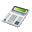Loan calculatorThis car loan calculator can calculate payments of a loan. Our soft calculator accepts: Loan amount: The total amount of money that you have borrowed. Percentage of Down Payment Loan term: The number of years you have to pay off your loan. Annual Interest Rate: The exact interest rate on your loan. This is deferent from the annual percentage rate or APR which is standardized method of calculating the cost of a loan, stated as a yearly rate which includes such items as interest, loan insurance, and certain points or credit costs.

# Car loan calculatorPurchase & Financing Information Loan amount: (In Dollars) Percentage of Down Payment: % Loan term: years Annual Interest Rate: % Explain Calculations: Show me the calculations and amortization

### Car payment calculatorYour dream of driving down a highway without a road map is not too far in the far-away future thanks to auto finance and this car payment calculator. This calculator is a good tool that gives you a reasonable approximation of the predictable monthly repayment sum for any obtainable vehicle loan.

Auto loan calculator